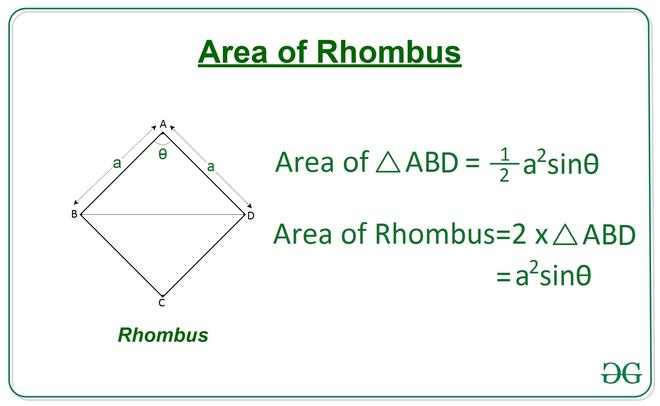# Find the area of rhombus from given Angle and Side length

• Last Updated : 07 Apr, 2021

Given two integers A and X, denoting the length of a side of a rhombus and an angle respectively, the task is to find the area of the rhombus.

A rhombus is a quadrilateral having 4 sides of equal length, in which both the opposite sides are parallel, and opposite angles are equal.

Examples:

Input: A = 4, X = 60
Output: 13.86

Input: A = 4, X = 30
Output: 8.0

Approach:For a Rhombus ABCD having the length of a side a and an angle x, the area of triangle ABD can be calculated using Side-Angle-Side property of triangle by the following equation:

Area of Triangle ABD = 1/2 (a2) sin x
Area of Rhombus ABCD will be double the area of ABD triangle.

Therefore, Area of Rhombus ABCD = (a2) sin xBelow is the implementation of the above approach:

## C++

 `// C++ Program to calculate``// area of rhombus from given``// angle and side length``#include ``using` `namespace` `std;` `#define RADIAN 0.01745329252``// Function to return the area of rhombus``// using one angle and side.``float` `Area_of_Rhombus(``int` `a, ``int` `theta)``{``    ``float` `area = (a * a) * ``sin``((RADIAN * theta));``    ``return` `area;``}` `// Driver Code``int` `main()``{``    ``int` `a = 4;``    ``int` `theta = 60;` `    ``// Function Call``    ``float` `ans = Area_of_Rhombus(a, theta);` `    ``// Print the final answer``    ``printf``(``"%0.2f"``, ans);``    ``return` `0;``}` `// This code is contributed by Rajput-Ji`

## Java

 `// Java Program to calculate``// area of rhombus from given``// angle and side length``class` `GFG{` `static` `final` `double` `RADIAN = ``0.01745329252``;``  ` `// Function to return the area of rhombus``// using one angle and side.``static` `double` `Area_of_Rhombus(``int` `a, ``int` `theta)``{``    ``double` `area = (a * a) * Math.sin((RADIAN * theta));``    ``return` `area;``}` `// Driver Code``public` `static` `void` `main(String[] args)``{``    ``int` `a = ``4``;``    ``int` `theta = ``60``;` `    ``// Function Call``    ``double` `ans = Area_of_Rhombus(a, theta);` `    ``// Print the final answer``    ``System.out.printf(``"%.2f"``, ans);``}``}` `// This code is contributed by Rajput-Ji`

## Python3

 `# Python3 Program to calculate``# area of rhombus from given``# angle and side length``  ` `import` `math ``  ` `# Function to return the area of rhombus``# using one angle and side. ``def` `Area_of_Rhombus(a, theta): ``  ` `    ``area ``=` `(a``*``*``2``) ``*` `math.sin(math.radians(theta))``  ` `    ``return` `area ``  ` `# Driver Code ``a ``=` `4``theta ``=` `60``  ` `# Function Call ``ans ``=` `Area_of_Rhombus(a, theta) ``  ` `# Print the final answer``print``(``round``(ans, ``2``))`

## C#

 `// C# Program to calculate``// area of rhombus from given``// angle and side length``using` `System;``class` `GFG{` `static` `readonly` `double` `RADIAN = 0.01745329252;``  ` `// Function to return the area of rhombus``// using one angle and side.``static` `double` `Area_of_Rhombus(``int` `a, ``int` `theta)``{``    ``double` `area = (a * a) * Math.Sin((RADIAN * theta));``    ``return` `area;``}` `// Driver Code``public` `static` `void` `Main(String[] args)``{``    ``int` `a = 4;``    ``int` `theta = 60;` `    ``// Function Call``    ``double` `ans = Area_of_Rhombus(a, theta);` `    ``// Print the readonly answer``    ``Console.Write(``"{0:F2}"``, ans);``}``}` `// This code is contributed by Rajput-Ji`

## Javascript

 ``

Output:

`13.86`

Time Complexity: O(1)
Auxiliary Space: O(1)

My Personal Notes arrow_drop_up